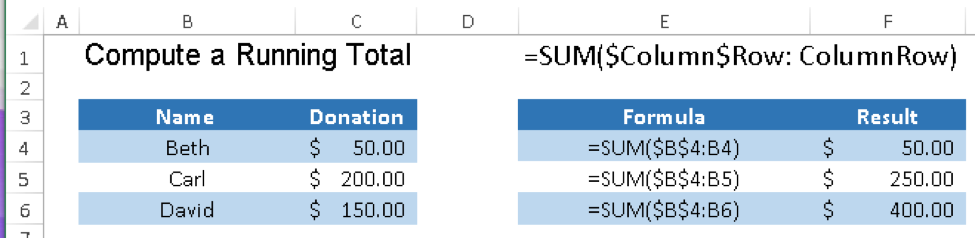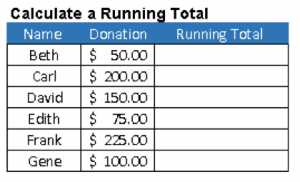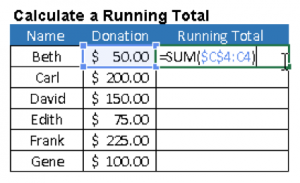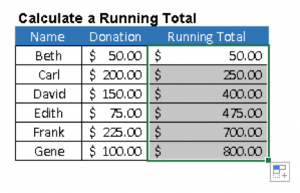### Calculate a Running Total in Excel & Google Sheets

This tutorial will teach you how to set up and calculate a running total with Excel.To calculate a running total, you’ll need to use the SUM function with a combination of absolute and relative references. Mixed references will allow you to create a growing total that is anchored to the initial value.Here is a list of people and the amount they have donated. You want to see howthe total donations grow as each individual contributes his or her donation. This increasing value is the running total.

## Running Total Formula

This is how to use the SUM formula: =SUM(\$Column\$Row:ColumnRow)

In the first row, the example use =SUM(\$C\$4:C4). This formula is telling Excel to add all the values from cell C4 to C4, which is only one cell and will give you the answer of \$50. As we copy this formula down, Excel will sum C4 to C5, C4 to C6, etc.## Absolute vs Relative Cell References

The \$ symbols in the formula freeze the cell reference (\$C\$4) in place. As you copy the formula down, the formula will always start with cell C4. This is called an absolute reference.

The second value in the formula (C4), does not have \$s and is therefore a relative reference. A relative reference will change when you copy the formula into the next row.
By creating a mixed cell reference like this, we can create a running total.